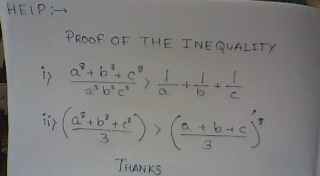helpgive me the total solutionNote by Superman Son
6 years, 4 months ago

This discussion board is a place to discuss our Daily Challenges and the math and science related to those challenges. Explanations are more than just a solution — they should explain the steps and thinking strategies that you used to obtain the solution. Comments should further the discussion of math and science.

When posting on Brilliant:

• Use the emojis to react to an explanation, whether you're congratulating a job well done , or just really confused .
• Ask specific questions about the challenge or the steps in somebody's explanation. Well-posed questions can add a lot to the discussion, but posting "I don't understand!" doesn't help anyone.
• Try to contribute something new to the discussion, whether it is an extension, generalization or other idea related to the challenge.
• Stay on topic — we're all here to learn more about math and science, not to hear about your favorite get-rich-quick scheme or current world events.

MarkdownAppears as
*italics* or _italics_ italics
**bold** or __bold__ bold

- bulleted
- list

• bulleted
• list

1. numbered
2. list

1. numbered
2. list
Note: you must add a full line of space before and after lists for them to show up correctly
paragraph 1

paragraph 2

paragraph 1

paragraph 2

> This is a quote
This is a quote
# I indented these lines
# 4 spaces, and now they show
# up as a code block.

print "hello world"
# I indented these lines
# 4 spaces, and now they show
# up as a code block.

print "hello world"
MathAppears as
Remember to wrap math in $$...$$ or $...$ to ensure proper formatting.
2 \times 3 $2 \times 3$
2^{34} $2^{34}$
a_{i-1} $a_{i-1}$
\frac{2}{3} $\frac{2}{3}$
\sqrt{2} $\sqrt{2}$
\sum_{i=1}^3 $\sum_{i=1}^3$
\sin \theta $\sin \theta$
\boxed{123} $\boxed{123}$

Sort by:

Diganta B., please don't forget to mention $\geq$ instead of $>$ in your question.

For the first one: Assume, by symmetry, $a \geq b \geq c$. Then, by Rearrangement inequality on the three sets $a^{2},b^{2},c^{2}$ , $a^{3},b^{3},c^{3}$ and $a^{3},b^{3},c^{3}$, we get

$a^{2}a^{3}a^{3}+b^{2}b^{3}b^{3}+c^{2}c^{3}c^{3}\geq a^{2}b^{3}c^{3}+a^{3}b^{2}c^{3}+a^{3}b^{3}c^{2}$

which gives $a^{8}+b^{8}+c^{8} \geq a^{3}b^{3}c^{3} (\frac {1}{a} + \frac {1}{b} + \frac {1}{c})$ And finally

$\frac {a^{8}+b^{8}+c^{8}}{a^{3}b^{3}c^{3}} \geq (\frac {1}{a} + \frac {1}{b} + \frac {1}{c})$

For the second question:

$\frac {a^{8}+b^{8}+c^{8}}{3} > (\frac {a+b+c}{3})^{8}$ is the same as

$(\frac {a^{8}+b^{8}+c^{8}}{3})^{\frac {1}{8}} > \frac {a+b+c}{3}$ and this nothing but the generalized mean with $M_{8}(a,b,c) > M_{1}(a,b,c)$. I hope you know about the generalized mean inequality.

Equality in both cases holds iff $a=b=c$

- 6 years, 4 months ago

thanks

- 6 years, 4 months ago

could you type these up? no offense, but i can't read any of the exponents

- 6 years, 4 months ago

exponent is 8

- 6 years, 4 months ago

use AM-GM inequality

- 6 years, 4 months ago

- 6 years, 4 months ago

sorry lah... ok I'll try shortly

- 6 years, 4 months ago

AM GM means Arithmetic mean and geometric mean...and there is an proved inequality for that...

- 6 years, 4 months ago

i know that

- 6 years, 4 months ago

I think the second one is Titu's Lemma...

- 6 years, 4 months ago

i. By AM-GM,

$\frac28a^8+\frac38b^8+\frac38c^8\geq a^2b^3c^3\\ \frac28b^8+\frac38c^8+\frac38a^8\geq b^2c^3a^3\\ \frac28c^8+\frac38a^8+\frac38b^8\geq c^2a^3b^3$

Adding these three inequalities and dividing by $a^3b^3c^3$ gives the desired inequality.

ii. This is a special case of the power-mean inequality on three variables.

- 6 years, 4 months ago

Please type this up. I can't read it clearly.

- 6 years, 4 months ago

1st one is obvious by weighted AM-GM. Second one is obvious by Jensen's inequality.

- 6 years, 4 months ago

explain clearly

- 6 years, 4 months ago

Please don't, it is best for people to figure it out for themselves! By giving away more we learn less.

- 6 years, 4 months ago

I thought we weren't supposed to put boring homework on this forum...

- 6 years, 4 months ago

it is not my homework guys

- 6 years, 4 months ago

its an example from a book called excursion in mathemay=tics

- 6 years, 4 months ago

Ya! It is a famous book in India and a must for the basics.

- 6 years, 4 months ago

I am sure you did not see the problems properly.

- 6 years, 4 months ago

I thought we were't supposed to bring homework on this forum. boring or otherwise.

- 6 years, 4 months ago